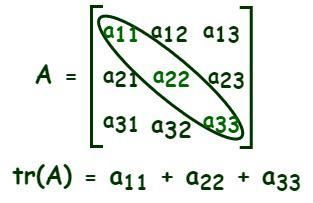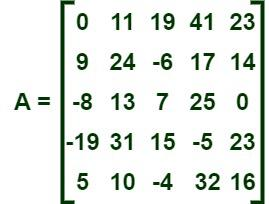Open in App
Not now

# Trace of a Matrix

• Last Updated : 08 Jan, 2023

A matrix is defined as a rectangular array of numbers that are arranged in rows and columns. The size of a matrix can be determined by the number of rows and columns in it. A matrix is said to be an “m by n” matrix when it has “m” rows and “n” columns and is written as an “m × n” matrix. For example, if a matrix has three rows and four columns, then the order of the matrix is “3 × 4.” We have different types of matrices, such as rectangular, square, triangular, symmetric, etc.

## Square Matrix

Before learning the concept of the trace of a matrix, we need to know about a square matrix. A square matrix is defined as a matrix that has an equal number of rows and columns. For example, if the order of a square matrix is “3 × 3,” then it has three rows and three columns.

Square Matrix of order “2 × 2”

A =Square matrix of order “3 × 3”

B =## What is Trace of a Matrix?

Trace of a matrix is defined as the sum of the principal diagonal elements of a square matrix. It is usually represented as tr(A), where A is any square matrix of order “n × n.” Let us consider a square matrix of order “3 × 3,” as shown in the figure given below, a11, a12, a13,…, a32, a33 are the entries of the given matrix A. Now, the trace of matrix “A” is equal to the sum of its principal diagonal elements, i.e., a11, a22, and a33.If A is a square matrix of order “n × n,” then the trace of matrix A is equal to the sum of the main diagonal elements.

tr(A) = a11 + a22 + a33 + …+ ann

## Properties of Trace of a Matrix

The following are some important properties of a trace of a matrix. Let us consider two square matrices A and B of the same order.

• The sum of the traces of the matrix A and the matrix B is equal to the trace of the matrix that is obtained by the sum of the matrices A and B.

tr(A) + tr(B) = tr (A + B)

• The trace of a given matrix and its transpose are the same.

tr(A) = tr (AT)

• If A is any square matrix of order “n × n” and k is a scalar, then

tr(kA) = k Tr(A)

• If A is a matrix of order “m × n” and B is a matrix of order “n × m,” then the trace of AB is equal to the trace of BA.

tr (AB) = tr (BA)

The above statement is true if both AB and BA are defined.

•  The trace of an identity matrix of order “n × n” is n.

tr(In) = n

• The trace of a zero or null matrix of any order is zero.

tr(O) = 0

Also, Check

## Solved Examples on Trace of a Matrix

Example 1: Prove that the trace of an identity matrix of order “3 × 3” is 3.

Solution:

Let us consider an identity matrix of order “3 × 3” to prove the trace of an identity matrix of order “3 × 3” is 3.

I3 =We know that,

tr(A) = a11 + a22 + a33

tr(A) = 1 + 1 + 1 =3

Hence, proved.

Example 2: Calculate the trace of the matrix given below.

B =Solution:

From the given matrix,

b11 = 1, b22 = 11, b33 = −5, and b44 = −4.

We know that,

tr(A) = b11 + b22 + b33 + b44

= 1 + 11 + (−5) + (−4)

= 12 −5 −4 = 12 − 9 = 3

Thus, the trace of the given matrix B is 3.

Example 3: Calculate the trace of the matrix given below.Solution:

From the given matrix,

a11 = 0, a22 = 24, a33 = 7, a44 = −5, and a55 = 16.

We know that,

tr(A) = a11 + a22 + a33 + a44 + a55

= 0 + 24 + 7 + (−5) + 16

= 47 −5 = 42

Thus, the trace of the given matrix A is 42.

Example 4: If R = P + Q, then prove that tr(R) = tr(P) + tr(Q), where “P, Q, and R” are square matrices of order “2 × 2”

Solution:

Let P =Q =R = P + QNow, tr(R) = p11 + q11 + p22 + q22

tr(R) = p11 + p22 + q11 + q22

tr(P) = p11 + p22

tr(Q) = q11 + q22

tr(P) + tr(Q) = p11 + p22 + q11 + q22

tr(P) + tr(Q) = tr(R)

Hence, proved.

## FAQs on Trace of a Matrix

Question 1: What is a square matrix?

A square matrix is defined as a matrix that has an equal number of rows and columns. For example, if the order of a square matrix is “3 × 3,” then it has three rows and three columns.

Question 2: What is meant by the trace of a matrix?

The trace of a matrix is defined as the sum of the principal diagonal elements of a square matrix. It is usually represented as tr(A), where A is any square matrix of order “n × n.”

Question 3: What is the trace of a null matrix?

The trace of a zero or null matrix of any order is zero, i.e., tr(O) = 0.

Question 4: What is the trace of an identity matrix of order n?